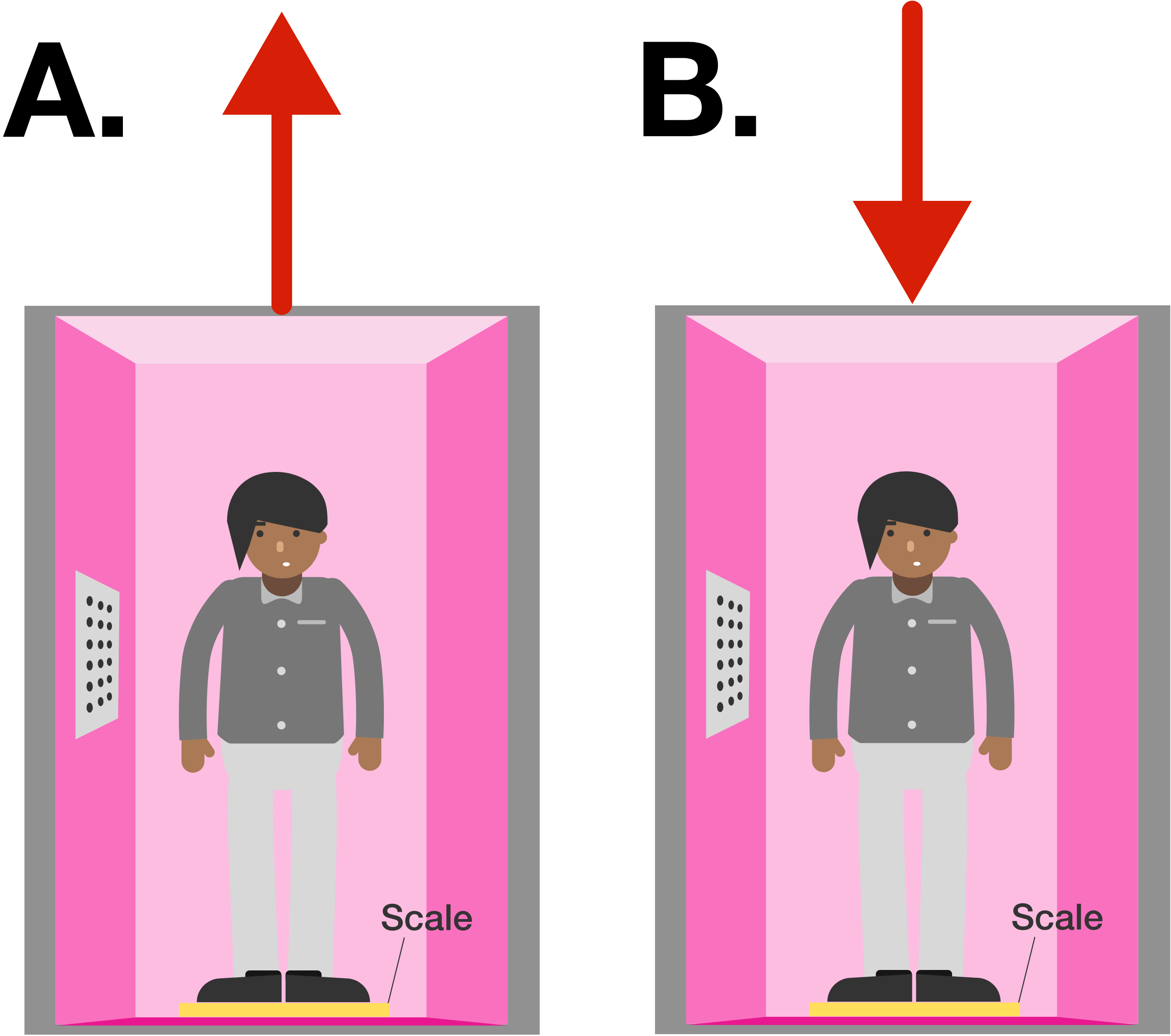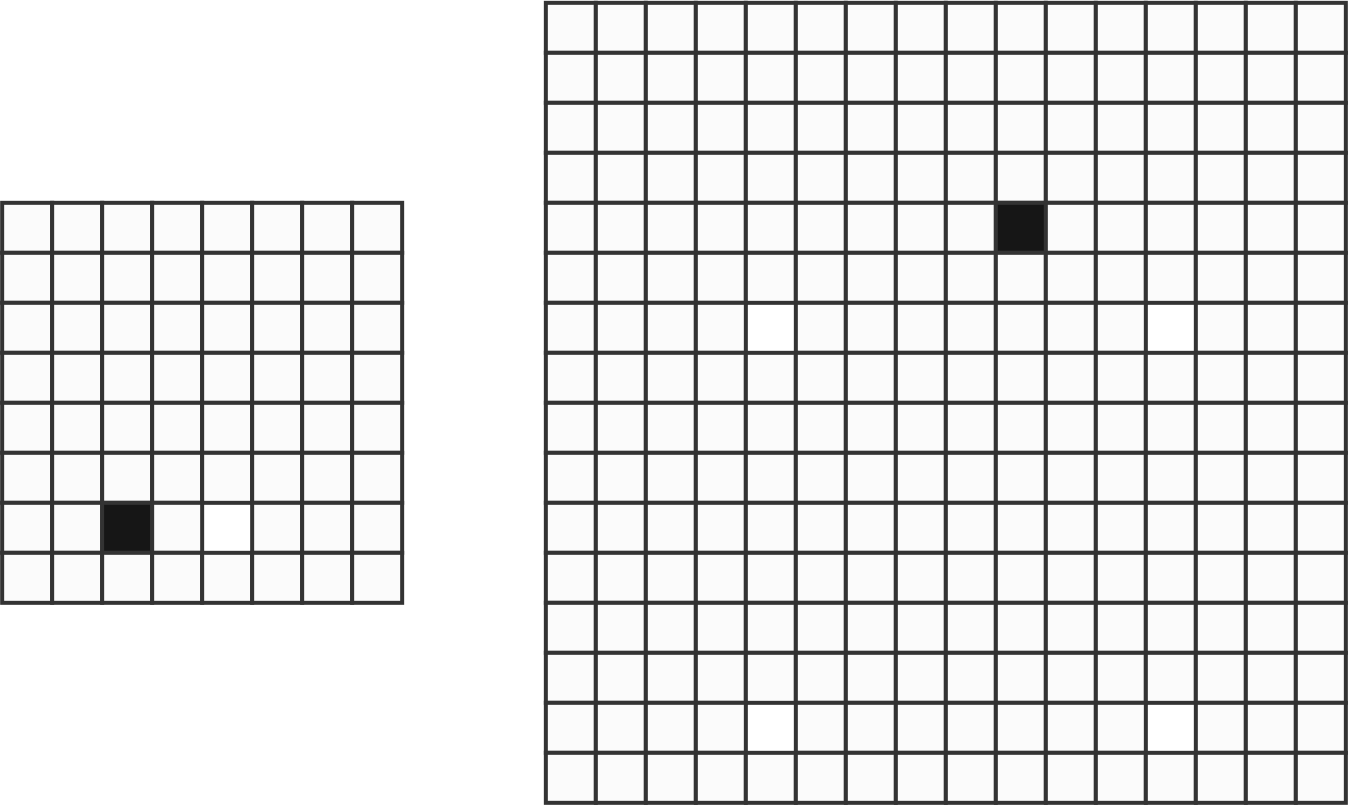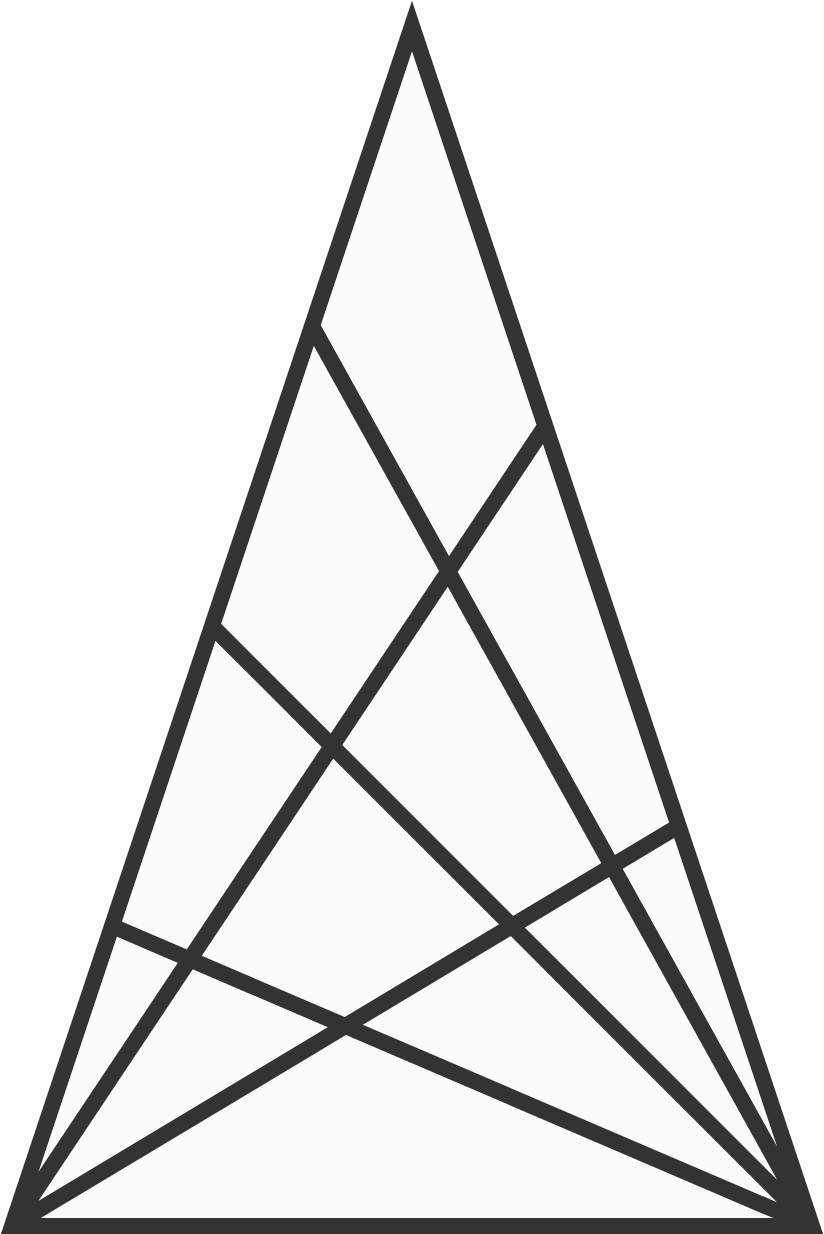# Problems of the Week

Contribute a problem

# 2018-08-13 Basic

Pam normally weighs $\SI{60}{\kilo\gram},$ but one day she measured her weight in an elevator as $\SI{70}{\kilo\gram}.$

Can we say for sure whether the elevator was moving up or down at the moment she measured her weight?The red arrows indicate the direction of motion.

Hint: Consider not just the velocity, but the acceleration of the elevator. Do velocity and acceleration always have the same direction?

$\begin{array}{cccc} & \color{#D61F06}A & \color{#D61F06}A & \color{#D61F06}A \\ \times & & & \color{#D61F06}A \\ \hline 3 & \color{#3D99F6}B & \color{#3D99F6}B & \color{#D61F06}A \end{array}$

$\color{#D61F06}A$ and $\color{#3D99F6}B$ are digits. What is ${\color{#3D99F6}B}?$

Is there a prime number $p$ that satisfies this inequality?

$2018!+2 \le p \le 2018!+2018$

Note: $2018!$ is the factorial of 2018. That is, $2018!=1 \times 2 \times 3 \times \cdots \times 2016 \times 2017 \times 2018.$

This $4\times 4$ grid of unit squares is tiled with L-shaped pieces of area 3, with one square blacked out.Now, the side length $4=2^2$ of this square grid is extended to a larger power of 2, as illustrated below, and again one of the squares is blacked out.

Can you always tile this grid with L-shaped pieces of area 3, no matter where the black square is?The grid can have side length 8, 16, 32, or any power of 2. One square is blacked out in each grid.How many quadrilaterals are drawn in this figure?

Hint: Be sure to count both convex and concave quadrilaterals.

×